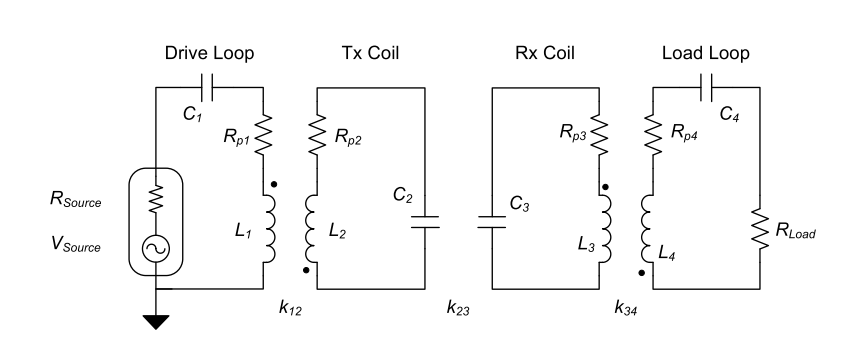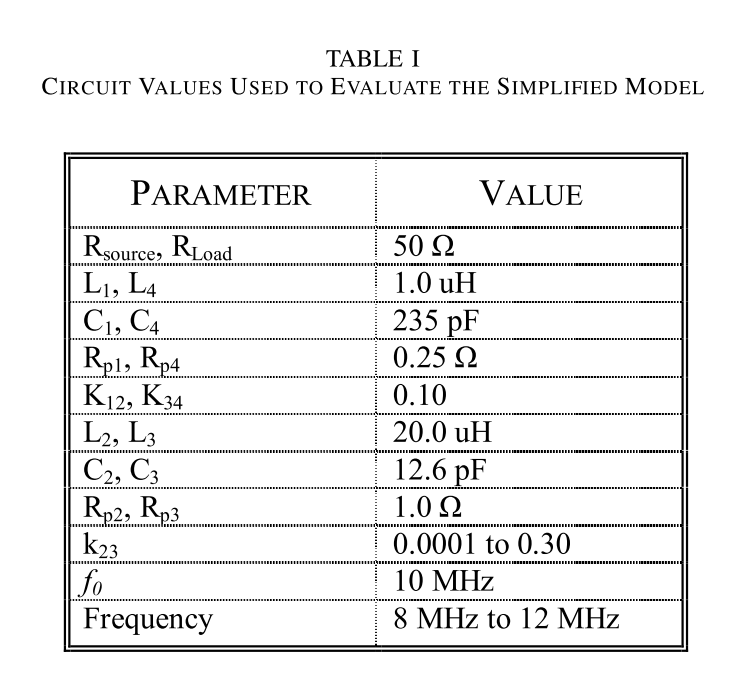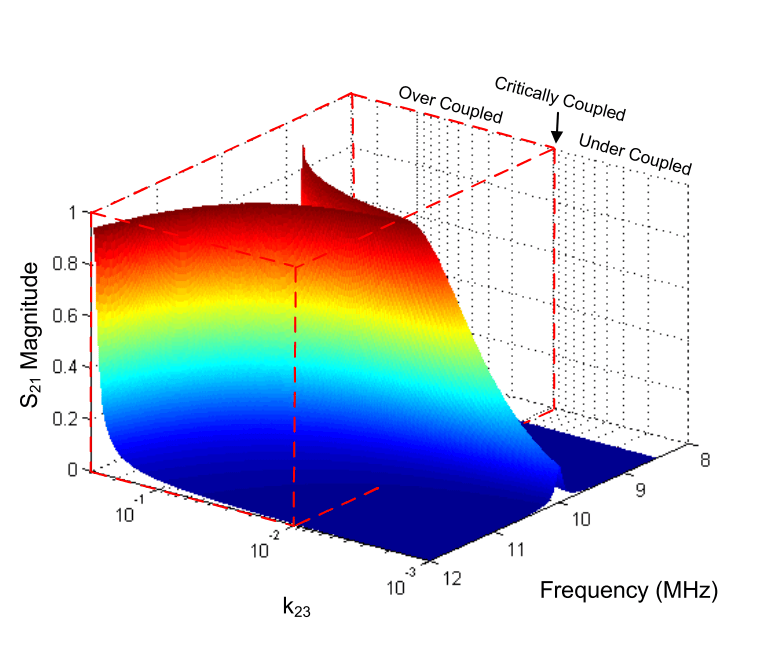LTSpice can be used to calculate the efficiency and other parameters in wireless power transfer (WPT).

First, We build the equivalent circuit diagram of the WPT system below. The figure and data came from the following paper.

Sample, A. P., Meyer, D. a., & Smith, J. R. (2011). Analysis, experimental results, and range adaptation of magnetically coupled resonators for wireless power transfer. IEEE Transactions on Industrial Electronics, 58(c), 544–554. http://doi.org/10.1109/TIE.2010.2046002The related parameters of each lumped circuit elements are shown at the table below.

There are four inductors in this system. L1 and L4 show the feed coils.  L2 and L3 show the resonant coils in each TRX and RCV coil.

The resonant frequency is set to be 10 MHz with the help of C2 and C3.

In this analysis, the coupling coefficient K is important. K is defined the ratio of mutual inductance over each self inductance of coil.

The distance of the resonant coil is directly related to the K23 which shows the coupling between L2 and L3.

K12 and K34 are fixed into 0.1 here.We build the schematics of circuit using LTSpice.  You can see the following link how to setting LTSpice for calculating S-parameters.

wirelesspower_spice

S-parameters with LTSpiceRun the simulation and plot the S21 parameter.

S21 can be expressed by this following equation.

S21 = 2* Vload/Vsource * sqrt(R_source/R_Loda)

This is the output plot of the S21.## 1件のコメント

1. […]  http://wireless-square.com/2016/11/02/wireless-power-transfer-analysis-using-ltspice/ […]

このサイトはスパムを低減するために Akismet を使っています。コメントデータの処理方法の詳細はこちらをご覧ください